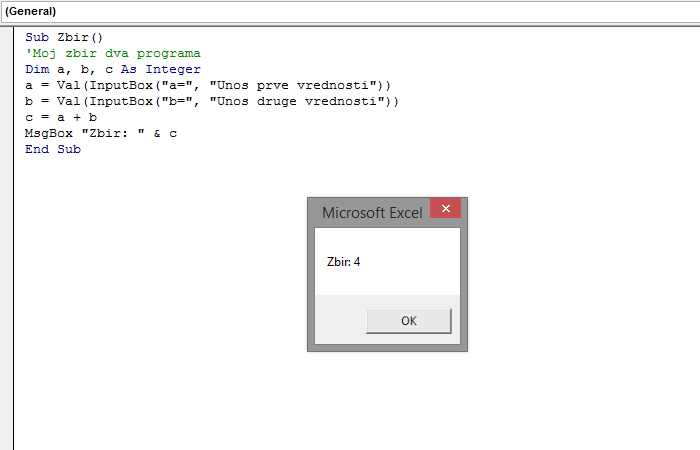# MsgBox and InputBox

MsgBox is an internal VBA procedure (method) used to display content within a dialog window that appears on the screen. It can be used to display the operand that we have directly transmitted or the values ​​of the variables that are calculated within the subroutine. InputBox is a function that we use to enter values ​​that we will later use to calculate the expression.

The simplest way to use MsgBox is if we directly forward a parameter to it. For example:

MsgBox “Hello, World!”

If we have previously declared a variable and have performed a calculation operation, we will write the following code:

Dim as As Integer
a = 2 + 2
MsgBox a

MsgBox can also contain multiple operands connected in one using the “&” sign. For example, if we want to display text that goes in two rows, we will write a program:

MsgBox “First” & vbNewLine & “Other”

In the middle of this expression there is an internal constant vbNewLine that is used to switch output to a new line.

MsgBox can also act as a function. In this case, in addition to the value displayed, it is also possible to define the window type, text in the title bar, the location of the help file, etc. See example:

Dim, b, c As Integer
a = 2
b = 2
c = MsgBox (a + b, vbOKOnly, “Number two numbers”)

InputBox returns Variant values, so it’s convenient to declare a variable by assigning the specified type to it.

Sub Sum ()
‘My sum of two programs
Dim, b, c As Integer
a = Val (InputBox (“a =”, “Input of the first value”))
b = Val (InputBox (“b =”, “Input of another value”))
c = a + b
MsgBox “Sum:” & c
End Sub

InputBox treats entered values ​​as strings, so if we want to execute some arithmetic operations over them, it would be useful to first convert them to a large value using the Val function.